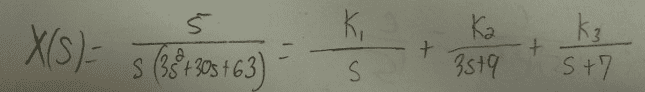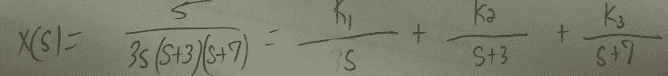# Partial Fraction Expansion

btbam91
For PFE, can a denominator variables with coefficient of something other than 1, or does it have to be 1?

For Example, can I have a term A/(3x+9)?

It's been years since I've dealt with this and don't quite remember if this was a rule or not.

Thanks!

EDIT: This is in terms of taking the inverse laplace transform later.

Last edited:

## Answers and Replies

btbam91
Just to put things into perspective, is the first image ok or do I have to follow what I did in the second image?

#### Attachments

Staff Emeritus
Homework Helper
Gold Member
For PFE, can a denominator variables with coefficient of something other than 1, or does it have to be 1?

For Example, can I have a term A/(3x+9)?

It's been years since I've dealt with this and don't quite remember if this was a rule or not.

Thanks!

EDIT: This is in terms of taking the inverse Laplace transform later.

You can do it either way. But why would you not factor out the 3?btbam91
It's just been a while since I've done these. For some reason I thought it was a rule that the coefficients had to be 1 or something.

In my 2nd image, is it set up correctly? Where the 3 remains on the left but the K1/s should not be K1/3s?

Staff Emeritus
$\displaystyle \frac{5}{s(3s^2+30s+63)}= \frac{1}{3}\left(\frac{k_1}{s}+\frac{k_2}{s+3}+ \frac{k_3}{s+7}\right)$​